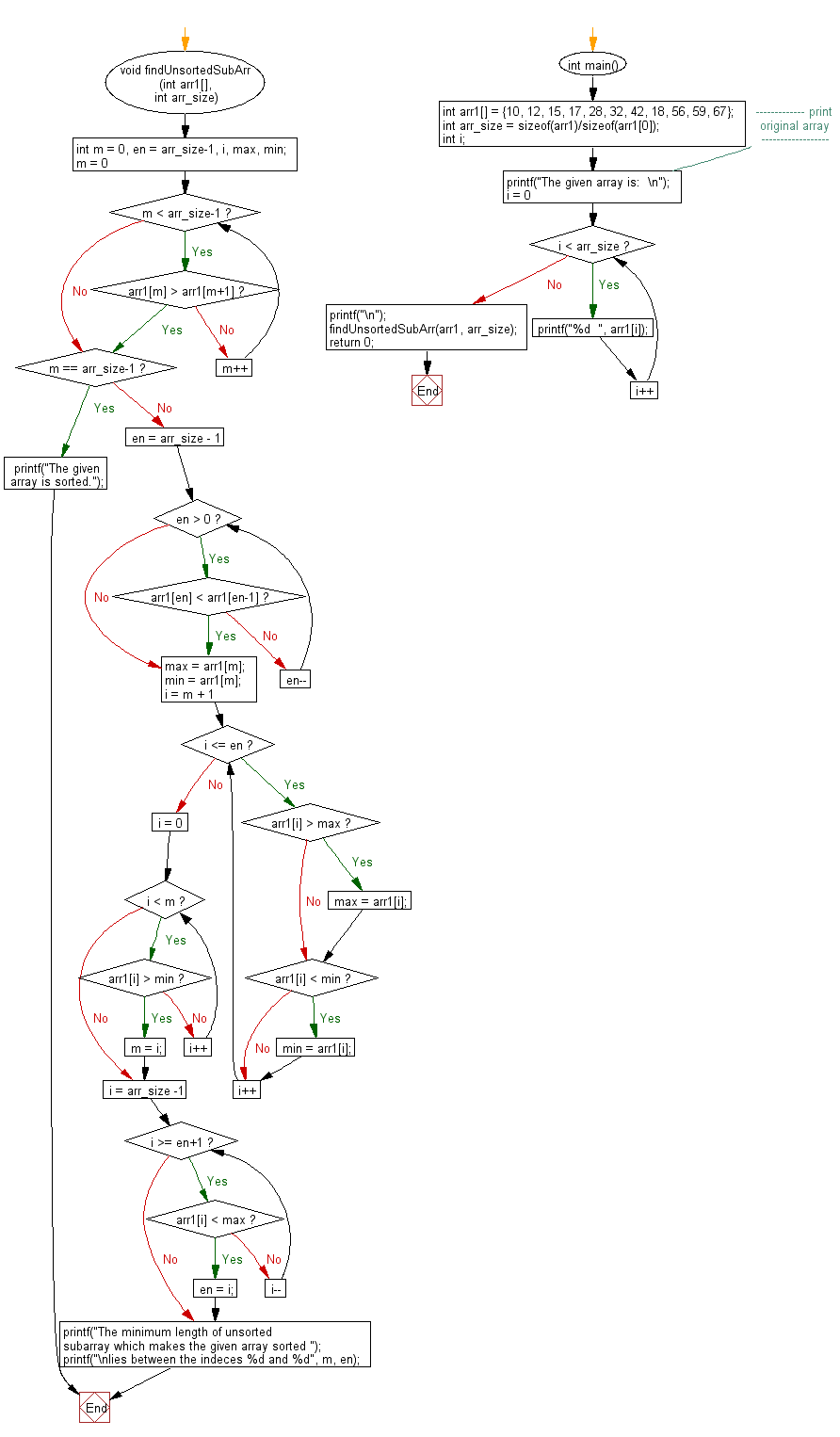﻿ C exercises: Find the minimum length of subarray such that, sorting this subarray makes the whole array sorted - w3resource# C Exercises: Find the minimum length of subarray such that, sorting this subarray makes the whole array sorted

## C Array: Exercise-91 with Solution

Given an unsorted array of specific size. Write a program in C to find the minimum length of subarray such that, sorting this subarray makes the whole array sorted.

Sample Solution:

C Code:

``````#include<stdio.h>

void findUnsortedSubArr (int arr1[], int arr_size)
{
int m = 0, en = arr_size-1, i, max, min;

for (m = 0; m < arr_size-1; m++)
{
if (arr1[m] > arr1[m+1])
break;
}
if (m == arr_size-1)
{
printf("The given array is sorted.");
return;
}

for(en = arr_size - 1; en > 0; en--)
{
if(arr1[en] < arr1[en-1])
break;
}

max = arr1[m]; min = arr1[m];
for(i = m + 1; i <= en; i++)
{
if(arr1[i] > max)
max = arr1[i];
if(arr1[i] < min)
min = arr1[i];
}

for( i = 0; i < m; i++)
{
if(arr1[i] > min)
{
m = i;
break;
}
}

for( i = arr_size -1; i >= en+1; i--)
{
if(arr1[i] < max)
{
en = i;
break;
}
}
printf("The minimum length of unsorted subarray which makes the given array sorted ");
printf("\nlies between the indeces %d and %d", m, en);
return;
}

int main()
{
int arr1[] = {10, 12, 15, 17, 28, 32, 42, 18, 56, 59, 67};
int arr_size = sizeof(arr1)/sizeof(arr1);
int i;
//------------- print original array ------------------
printf("The given array is:  \n");
for(i = 0; i < arr_size; i++)
{
printf("%d  ", arr1[i]);
}
printf("\n");
//-----------------------------------------------------------
findUnsortedSubArr(arr1, arr_size);
return 0;
}
```
```

Sample Output:

```The given array is:
10  12  15  17  28  32  42  18  56  59  67
The minimum length of unsorted subarray which makes the given array sorted
lies between the indeces 4 and 7
```

Pictorial Presentation:Flowchart:C Programming Code Editor:

Improve this sample solution and post your code through Disqus.

﻿

## C Programming: Tips of the Day

Where is the C auto keyword used?

auto is a modifier like static. It defines the storage class of a variable. However, since the default for local variables is auto, you don't normally need to manually specify it.

Ref : https://bit.ly/3yzwC9r